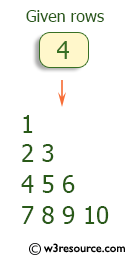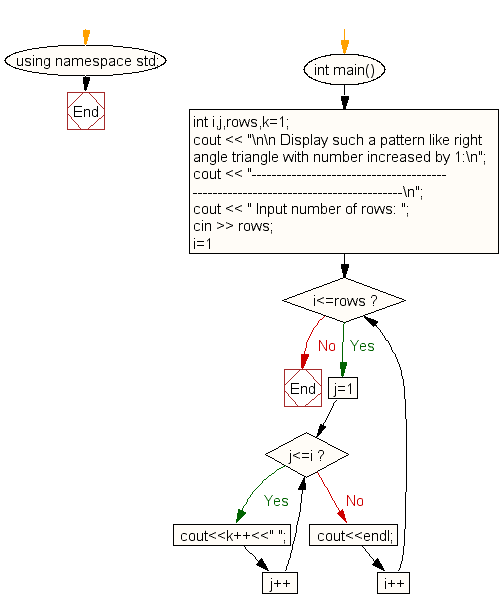﻿ C++ : Right angle triangle pattern with number increase by 1# C++ Exercises: Display the pattern like right angle triangle with number increased by 1

## C++ For Loop: Exercise-38 with Solution

Write a program in C++ to make such a pattern like right angle triangle with number increased by 1.

Pictorial Presentation:Sample Solution:-

C++ Code :

``````#include <iostream>
#include <string>
using namespace std;

int main()
{
int i,j,rows,k=1;
cout << "\n\n Display such a pattern like right angle triangle with number increased by 1:\n";
cout << "---------------------------------------------------------------------------------\n";
cout << " Input number of rows: ";
cin >> rows;
for(i=1;i<=rows;i++)
{
for(j=1;j<=i;j++)
cout<<k++<<" ";
cout<<endl;
}
}
``````

Sample Output:

``` Display such a pattern like right angle triangle with number increased by 1:
---------------------------------------------------------------------------------
Input number of rows: 4
1
2 3
4 5 6
7 8 9 10
```

Flowchart:C++ Code Editor:

Contribute your code and comments through Disqus.

What is the difficulty level of this exercise?

﻿